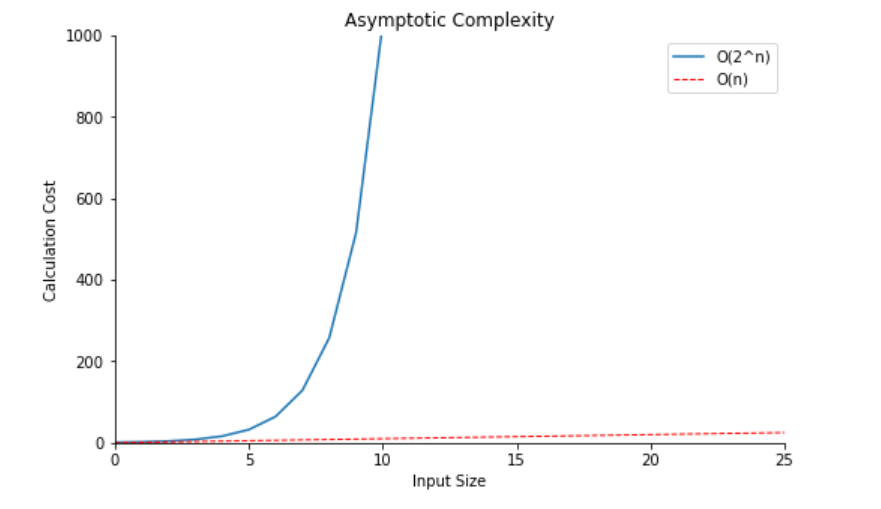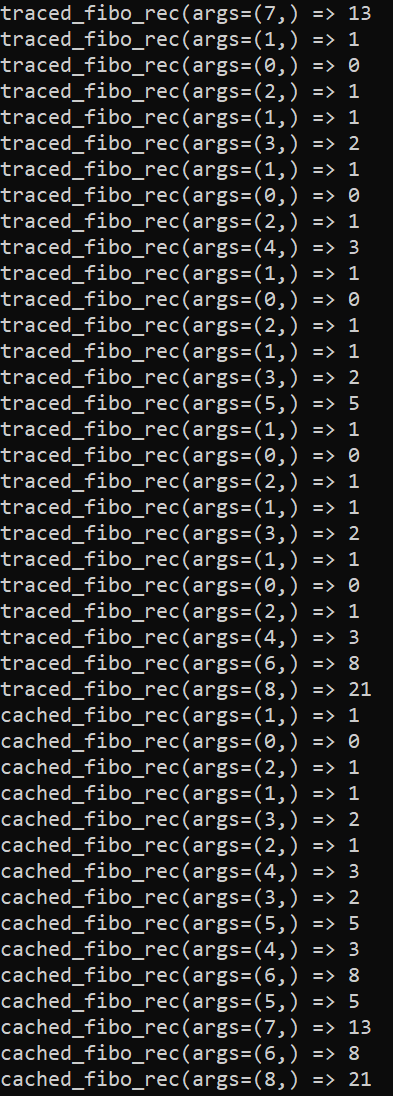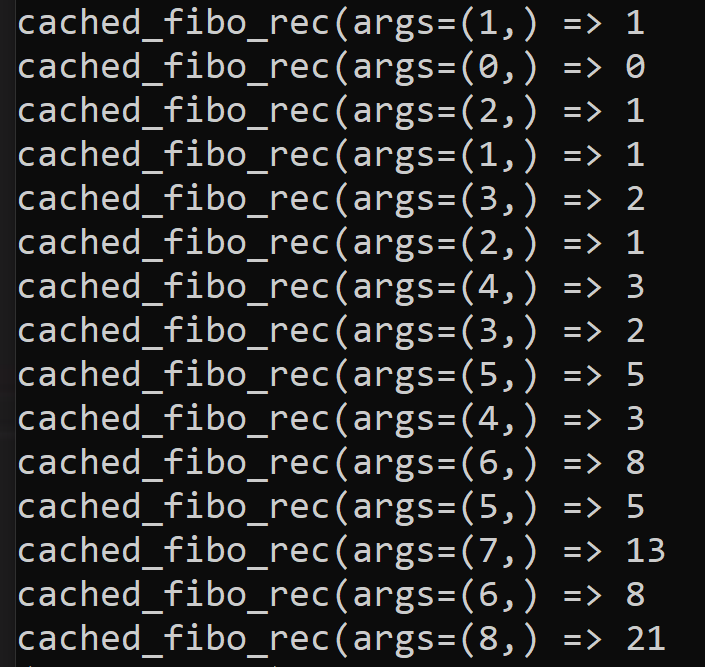#### Boost the performance of recursive functions with memoizationPhoto by Florian Krumm on Unsplash

### What is Memoization?

Memoization is a type of caching that stores the result of a deterministic function. More specifically, memoization is an optimization technique used to accelerate programs by storing the results of function calls and returning the cached result when redundant inputs arise.

In other words, memoization prevents a program from running the same calculation twice.

Let’s see this behavior with an artificially slow Python function.

When we run slow_func.py we get the following output:

Notice that there are two redundant calculations; each one adds a second to our execution time. Now let us write a new version of this function to illustrate memoization.

On line three, we have added an empty dictionary to serve as a cache. Now on lines 7–8, we check the cache to see if slow_func has seen its current input before, returning the result if present. Line 13 stores any new number and it's square in the cache.

Now when we run memoized_slow_func.py our output looks like this:

Notice that the calculation step has been skipped for the two redundant inputs, effectively cutting our runtime in half. Now that you understand the purpose of memoization, let’s move on to some more complicated examples.

### What is the Fibonacci Sequence?Photos by Paul Milasan and Aaron Burden on Unsplash

The Fibonacci Sequence is the series of numbers:

0, 1, 1, 2, 3, 5, 8, 13, 21, 34, …

The next number is found by adding up the two numbers before it.

f(n) = f(n-1) + f(n-2)

An important characteristic of the sequence is that the ratio between any number and the previous one in the series tends towards a well-defined value: 1.618… This is the golden ratio or golden section, φ (Phi), that frequently occurs naturally.

Observing the geometry of plants, flowers or fruit, it is easy to recognize the presence of recurrent structures and forms. The Fibonacci sequence, for example, plays a vital role in phyllotaxis, which studies the arrangement of leaves, branches, flowers or seeds in plants.

For our purposes, the Fibonacci sequence is significant because it can be calculated recursively. Recursive functions can become very computationally expensive as their input size increases.

#### Fibonacci in Python

A Fibonacci number can be calculated iteratively:

Or recursively:

The iterative calculation will proceed from the beginning of the Fibonacci sequence, counting up until the input index is reached.

The recursive calculation mimics the mathematical notation and, in doing so, recursively calls itself. This means that for every value passed to fibo_rec, two calls to the function are made. These calls can also spawn more calls to the function. Therefore this is an expensive calculation.

Let’s see how the iterative and recursive functions perform with and without memoization.

### Comparing Computation Speeds

The timing and tracing decorators we use in this section(@tracefunc and @timefunc) can be found in this article.

A Deeper Dive into Decoration

Before we delve into the performance of each of our functions, let’s review the language associated with asymptotic time complexity.

#### Asymptotic complexity

Asymptotic computational complexity is the usage of asymptotic analysis to estimate the computational complexity of algorithms and computational problems, commonly associated with “big-O” notation.

In other words, using asymptotic analysis, we can get an idea about the performance of the algorithm based on the input size. In doing so, we should find the relationship between the run time and input size, expressed as “O” notation.

Our Fibonacci calculations are O(n) and O(2^n).Asymptotic Complexity or Big-O notation

#### Recursive Fibonacci

Recall that this is our expensive calculation. Its asymptotic complexity is O(2^n).

Here is a trace of traced_fibo_rec(8). Notice how many redundant inputs there are and how many calls to the function must be completed to return an output. O(2^n) means 2⁸ or 256 calls to fibo_rec.Trace of fibo_rec →Lots of computing

The call fibo_rec(8) was calculated in a little over one-thousandth of a second.

#### The issue with recursive functions: recall depth

When you provide a large input to a recursive function in Python, you will get an error. This is to avoid a stack overflow. The Python interpreter limits the recursion limit so that infinite recursions are avoided.

It's worth mentioning that you can alter the maximum recursion depth limit with the sysmodule.

#### Memoized Recursive Fibonacci

Now let’s apply memoization to our expensive calculation.

Here is the trace for cached_fibo_rec(8).Less computing

Notice how many fewer calls to the function there are! This results in a much faster execution time.

The recursive Fibonacci calculation is 133x faster with the cache. This is because our function only has to calculate each output value once. When a redundant input arises, the function pulls the output from the cache instead of spawning another deep recursive stack!

By adding memoization to this function, we have changed its big-O notation from O(2^n) to O(n). This is a massive increase in the performance of the algorithm.

#### Iterative Fibonacci

The asymptotic complexity of this function O(n). This is because it loops through the Fibonacci sequence from zero to n.

We can see that the call fibo_iter(8) was calculated in well under one-ten-thousandth of a second. This function runs at essentially the same speed as our memoized recursive version because they have the same asymptotic complexity.

It's worth noting that the iterative Fibonacci algorithm can compute a larger sequence number than the recursive algorithm because of the maximum recursion depth limit mentioned earlier.

#### Memoized Iterative Fibbonacci

Lastly, let's apply memoization to our iterative function to see if there is a change in performance.

We see essentially the same execution time with or without a cache for this algorithm. This indicates that memoization might be useless for fast functions (functions with better O notation). Perhaps for functions like this, computing the output has the same computational cost as accessing a cached value. For very fast functions, the cache accessing can even be slower than calculating redundant values!

### Conclusion

Memoization is a useful method of caching to optimize the performance of expensive function calls with redundant inputs. In some cases, memoization can improve the asymptotic complexity of an algorithm This is common in recursive or dynamic programming algorithms. For Example, when we applied memoization to a recursive algorithm with O(2^n) complexity, we saw a massive performance boost. In this case, accessing a cache is much faster than repeating a calculation.

Memoization allows us to use the recursive Fibonacci algorithm. However, the iterative calculation is still superior.How to Accelerate Expensive Algorithms was originally published in Towards Data Science on Medium, where people are continuing the conversation by highlighting and responding to this story.